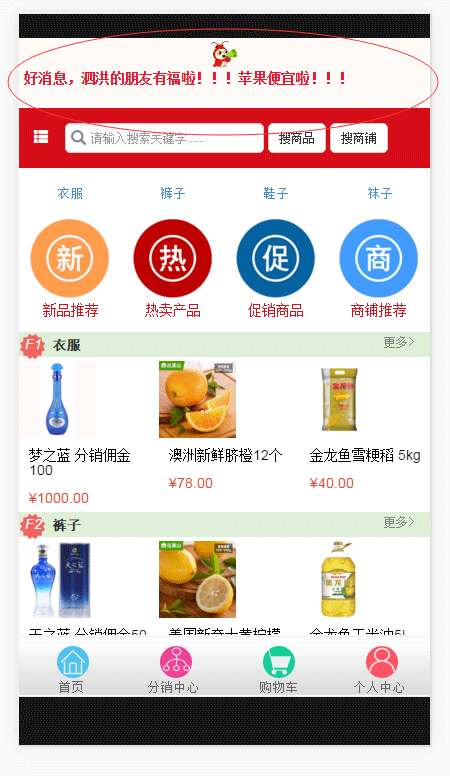# thinkPHP商城公告功能开发问题分析

更新时间：2016年12月01日 11:10:45   作者：牛逼的霍啸林我要评论1.定在头部

```position: fixed;
z-index: 999;
top: 0;
opacity:1;

```

2.ajax处理json数据

```// 获取商城公告
function getNotice() { // 获取公告函数
var res;
\$.ajax({
type: "POST",
url: "{sh::U('Store/Mall/ajaxGetNotice',array('mid'=>\$mid))}",
dataType:'json', // 设为json之后，就能够很好的处理获取的json数据，json.status
async: false,
success: function(json){
res = json;
}
});
return res;
}

```

3.最后加载，页面更好看。

```\$(document).ready(function(e) { // 主函数
// 获取公告
var action_name = "{sh::ACTION_NAME}"; // 页面使用thinkphp常量
var json = getNotice();
if ( action_name == 'index' && json.status == 1) { // 首页并且公告存在
\$(".top").css("margin-top", "70px"); // jquery设置css
\$(".main-sidebar").css("top" ,"70px");
var html = '';
\$.each(json.info, function(i, n){ // n为文本内容
html += "<li><strong>"+n.content+"</strong></li>"
});
\$(".top-notice").show();
\$('#notice ul').html(""+html);
\$('#notice').unslider(); // 轮播
}
});

```

4.获取sql语句的thinkphp处理

```// 获取公告
function ajaxGetNotice() {
if (IS_AJAX) {
\$this->mid;
// 获取有效的，且结束时间大于当前时间的，或者日期等于0的公告
\$mallNoticeModel = M('Mall_notice');
\$where['mall_id'] = \$this->mid;
\$where['status'] = 1;
\$where['endtime'] = array(array('eq',0),array('gt',time()), 'or') ;
//SELECT * from sh_mall_notice where mall_id = 9 and status = 1 and (endtime = 0 or endtime>1458354366);
\$notice = \$mallNoticeModel->where(\$where)->order('sort desc')->select();
if (!empty(\$notice)) {
\$this->ajaxReturn(array('status'=>'1','info'=>\$notice,'msg'=>"获取成功"),'JSON');
} else {
\$this->ajaxReturn(array('status'=>'2','info'=>\$notice,'msg'=>"公告不存在"),'JSON');
}
}
}

```
```\$where['endtime'] = array(array('eq',0),array('gt',time()), 'or') ;

```